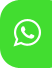## Product Detail## Ignou solved assignment for BCSL-045 Introduction to Algorithm design Lab

University  IGNOU
Service Type Solved Assignment
Course B.C.A
Semester
Short Name or Subject Code BCSL-045 Introduction to Algorithm design Lab
Product B.C.A of Solved Assignment (IGNOU)
Pattern
Price
Click to view price

Note: Answer all the questions in the assignment having 40 marks in total. 10 marks are for viva voce. You are required to write programs in C-language for all the problems , execute and show the results. You may use illustrations and diagrams to enhance the explanations. Please go through the guidelines regarding assignments given in the Programme Guide for the format of presentation. Make suitable assumption if necessary.

Q1. Write a program to compute 𝑋 𝑛 , where both 𝑋 and 𝑛 and integer numbers using left to right binary exponentiation algorithm.

Q2. Write a program to find the largest number in the five array using linear search algorithm. Calculate the total no of comparison operations and the number of times the loop will execute.

85 45 70 30 25 35 40 5 10 17

Q3. Write a program to traverse a graph using BFS. Apply this algorithm to the following graph and write the sequence of vertices to be travelled. Also calculate the number of times the loop(s) will execute.

Q4. Implement GCD (Greatest common divisor) problem using Euclid’s algorithm. Apply this algorithm to find the output of GCD of 225 and 15. Also calculate how many times the mod and equal operations will execute.

Q5. Implement and apply Kruskal’s algorithm to find a minimum cost spanning tree and test the result for the following graph:

Q6. Implement Karatsuba’s method using Divide & Conquer method to multiply two integer numbers. Test the result in multiplication of the following numbers and count the number of multiplication operations. 5 3 2 6 8 0 * 4 3 2 8 6

Q7. Draw a complete graph with 6 vertices. Write a C-Program to represent the graph using adjacency matrix and adjacency list.